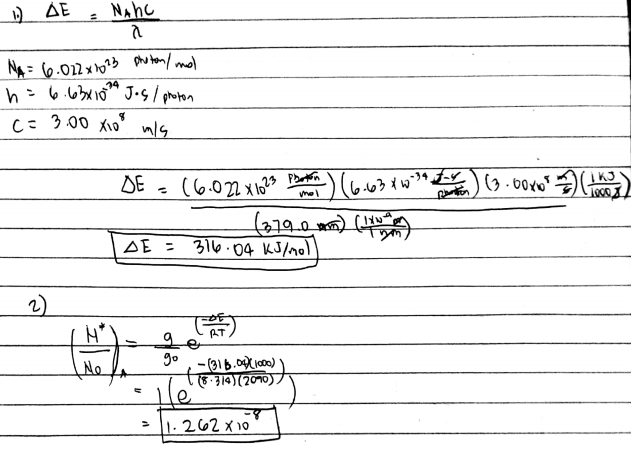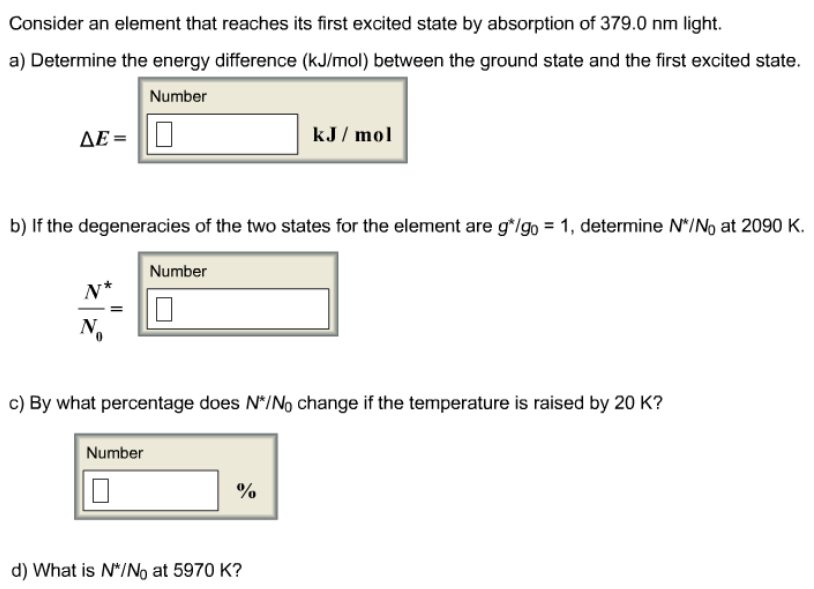# Problem: Consider an element that reaches its first excited state by absorption of 379.0 nm light.a) Determine the energy difference (kJ/mol) between the ground state and the first excited state.b) If the degeneracies of the two states for the element are g*/go = 1, determine N*/N_0 at 2090 Kc) By what percentage does N*/N_0 change if the temperature is raised by 20 K?d) What is N*/N_0 at 5970 K?

🤓 Based on our data, we think this question is relevant for Professor Sharma's class at UT.

###### FREE Expert Solution###### Problem Details

Consider an element that reaches its first excited state by absorption of 379.0 nm light.

a) Determine the energy difference (kJ/mol) between the ground state and the first excited state.

b) If the degeneracies of the two states for the element are g*/go = 1, determine N*/N_0 at 2090 K

c) By what percentage does N*/N_0 change if the temperature is raised by 20 K?

d) What is N*/N_0 at 5970 K?